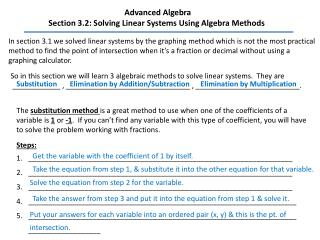# Advanced Algebra Section 3.2: Solving Linear Systems Using Algebra Methods - PowerPoint PPT PresentationDownload PresentationAdvanced Algebra Section 3.2: Solving Linear Systems Using Algebra Methods

Advanced Algebra Section 3.2: Solving Linear Systems Using Algebra MethodsDownload Presentation## Advanced Algebra Section 3.2: Solving Linear Systems Using Algebra Methods

- - - - - - - - - - - - - - - - - - - - - - - - - - - E N D - - - - - - - - - - - - - - - - - - - - - - - - - - -
##### Presentation Transcript

1. Advanced Algebra Section 3.2: Solving Linear Systems Using Algebra Methods In section 3.1 we solved linear systems by the graphing method which is not the most practical method to find the point of intersection when it’s a fraction or decimal without using a graphing calculator. So in this section we will learn 3 algebraic methods to solve linear systems. They are ____________ , _______________________________ , __________________________. Substitution Elimination by Addition/Subtraction Elimination by Multiplication The substitution methodis a great method to use when one of the coefficients of a variable is 1 or -1. If you can’t find any variable with this type of coefficient, you will have to solve the problem working with fractions. Steps: __________________________________________________________________ __________________________________________________________________ __________________________________________________________________ ___________________________________________________________________ ___________________________________________________________________ __________________ Get the variable with the coefficient of 1 by itself. Take the equation from step 1, & substitute it into the other equation for that variable. Solve the equation from step 2 for the variable. Take the answer from step 3 and put it into the equation from step 1 & solve it. Put your answers for each variable into an ordered pair (x, y) & this is the pt. of intersection.

2. Examples: Solve the system . 1. y = 2x 2x + 5y = -12 2x + 5(2x) = -12 y = 2x y = 2(-1) 2x + 10x = -12 12x = -12 y = -2 12 12 x = -1 (-1, -2) pt. of intersection

3. x + y = 6 3x + y = 15 x + y = 6 -y -y x = -y + 6 3(-y + 6) + y = 15 -3y + 18 + y = 15 -2y + 18 = 15 -18 -18 -2y = -3 -2 -2

4. Elimination by Addition/Subtraction We will use the _______________________________ method when the coefficients of either the x or y variables are opposites or the same number. EX: x –4y = 6 3x +4y = 10 ** The coefficients of the of the y variables are opposites of each other. Eliminate by Addition! 6x + 5y = 4 6x – 7y = -20 ** The coefficients of the x variablesare the samenumbers. Eliminate by Subtraction! Examples: Solve the linear system. 3. -3x + 4y = 12 3x - 4y = -18 Coefficients of the x & y variables are opposites, Eliminate by Addition. Lines are parallel.

5. 4. 3x + y = 6 4x + y = 7 The coefficients of the y variable are the same, Eliminate by Subtraction.

6. elimination by We will use our last algebraic method to solve a linear system which is ___________ ______________. multiplication Steps: Pick one of the variables that you want to elimination. Multiply one or both of the equations by a number so that the coefficients of one of the variables will be opposites or the same. 3. Solve the rest of the problem like we did with addition/subtraction method. Example: Solve the linear system. 5. 3x + 5y = 11 2x + 3y = 7 Solution:

7. 6. 3x – y = 2 x + 2y = 3

8. Lesson 3.2 Practice C in the packet: 1-19 all